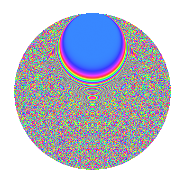# Properties

 Label 3150.2.ehLevel 3150 Weight 2 Character orbit eh Rep. character $$\chi_{3150}(113,\cdot)$$ Character field $$\Q(\zeta_{60})$$ Dimension 2880 Sturm bound 1440

# Related objects

## Defining parameters

 Level: $$N$$ = $$3150 = 2 \cdot 3^{2} \cdot 5^{2} \cdot 7$$ Weight: $$k$$ = $$2$$ Character orbit: $$[\chi]$$ = 3150.eh (of order $$60$$ and degree $$16$$) Character conductor: $$\operatorname{cond}(\chi)$$ = $$225$$ Character field: $$\Q(\zeta_{60})$$ Sturm bound: $$1440$$

## Dimensions

The following table gives the dimensions of various subspaces of $$M_{2}(3150, [\chi])$$.

Total New Old
Modular forms 11648 2880 8768
Cusp forms 11392 2880 8512
Eisenstein series 256 0 256

## Trace form

 $$2880q + 8q^{3} + O(q^{10})$$ $$2880q + 8q^{3} - 8q^{12} + 8q^{15} - 360q^{16} - 8q^{18} - 24q^{20} - 24q^{23} + 96q^{25} - 16q^{27} - 8q^{33} - 48q^{37} + 72q^{38} - 40q^{39} + 80q^{45} + 96q^{47} + 16q^{48} - 48q^{55} + 32q^{57} + 120q^{59} + 40q^{60} - 32q^{63} - 24q^{65} - 24q^{67} - 16q^{72} + 32q^{75} + 168q^{78} - 80q^{81} - 96q^{82} + 120q^{83} - 48q^{85} + 240q^{87} + 40q^{90} - 24q^{92} + 72q^{93} + 120q^{95} - 72q^{97} + O(q^{100})$$

## Decomposition of $$S_{2}^{\mathrm{new}}(3150, [\chi])$$ into newform subspaces

The newforms in this space have not yet been added to the LMFDB.

## Decomposition of $$S_{2}^{\mathrm{old}}(3150, [\chi])$$ into lower level spaces

$$S_{2}^{\mathrm{old}}(3150, [\chi]) \cong$$ $$S_{2}^{\mathrm{new}}(225, [\chi])$$$$^{\oplus 4}$$$$\oplus$$$$S_{2}^{\mathrm{new}}(450, [\chi])$$$$^{\oplus 2}$$$$\oplus$$$$S_{2}^{\mathrm{new}}(1575, [\chi])$$$$^{\oplus 2}$$

## Hecke Characteristic Polynomials

There are no characteristic polynomials of Hecke operators in the database